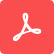# Symmetry

By Emerson Melo, Tom Chen and Xin Lei

In most FDTD simulations, field symmetries can be used to significantly reduce computation by 1/2, 1/4, or even 1/8. In this tutorial, you will learn how to set up symmetries based on the structure and source properties. As a practical example, we will show you how to properly set up symmetry conditions to excite the input waveguide of a photonic crystal slab polarization filter with both the fundamental TE and TM optical modes.

Share On:
Additional information: This Tutorial was updated in Aug 31, 2023Tidy3D GUI: Tutorial 10

In most FDTD simulations, you can use field symmetries to significantly reduce computation by 1/2, 1/4, or even 1/8, potentially saving significant time. Symmetry settings are located at the left sidebar. Properly defining symmetry conditions in FDTD simulations requires considering the geometries and positions of structures and sources within the simulation domain. Let's use the photonic crystal slab polarization filter, which you can find in the "Examples" folder, as an example. This structure itself exhibits mirror symmetries along planes that bisect the simulation domain normal to the x-, y-, and z-directions. However, when we consider the source plane's position, mirror symmetries are only present along the y- and z-directions.

To begin, please make a copy of this task and, in the "Boundary and Symmetry" panel, initially opt for "No symmetry". Go ahead and run the simulation.

Upon accessing the simulation results, you'll observe that the fundamental transverse electric (TE0) guided mode cannot propagate through the photonic crystal waveguide due to the bandgap effect.

On the other hand, the transverse magnetic (TM0) optical mode is allowed to propagate with high efficiency.

Let's set up the correct symmetry conditions in the x-, y-, and z-directions, starting with the TE0 injection case. To achieve this, in addition to considering the geometry of structures and source planes, we must consider the vectorial nature of the fields to correctly determine the type of symmetry.

As shown here, you can accomplish this by utilizing the mode solver to inspect the mode field distributions. At the symmetry plane normal to the y-direction, the TE0 mode conforms to a "PEC" like condition, meaning the field component normal to that plane (the "Ey" field) remains continuous across the interface. In contrast, the field component "Ez", which is tangential to the symmetry plane, is null at the interface.

Before setting up the symmetry, click the "Estimate FlexCredit" command to note that the simulation has about 10 million grid points when no symmetries are enforced. Then, click "Boundary and Symmetry" and choose "Odd, i.e. 'PEC' Symmetry" for the y-direction. After re-estimating the FlexCredit, you'll see the number of grid points reduced by half. Run the simulation and go to the post-run results. After setting up the y-symmetry, the field distribution remains the same as in the case with no symmetry. The "modal_flux" monitor will yield the same result as well. That will be more obvious if you zoom out on the amplitude axis. When uncertain about symmetry settings, you can always compare the results with cases without symmetries.

If you examine the TE0 magnetic field distributions, you'll observe that the magnetic field tangent to the z-symmetry plane is null. On the other hand, the component "Hz", normal to the symmetry plane, remains continuous, giving the mode a "PMC-like" condition at the z-symmetry plane.

Return to the symmetry definitions and set it to "Even, i.e. 'PMC' Symmetry." Enable the Symmetry visualization to double-check the symmetries applied to the simulation. The "Odd, i.e. 'PEC' Symmetry" condition is shown in red, whereas the "Even, i.e. 'PMC' Symmetry" is colored in blue. After clicking "Estimate FlexCredit," you'll see a four-fold reduction in grid points. Run the simulation and observe that the results remain consistent.

Now, let's explore how to set the symmetry to launch the TM0 mode and achieve a 4-fold reduction in simulation size as well. By examining the TM0 mode field distributions, you'll notice that this mode exhibits "PMC" symmetry in the y-direction and "PEC" symmetry in the z-direction. Set these symmetry options in the simulation and rerun it.

By implementing these symmetry conditions, the TE0 mode is filtered out, and the TM0 mode is now enabled to propagate throughout the waveguide, as evident from these results.

Lastly, in certain applications where the structure and sources are symmetric to all x-, y-, and z-directions, like in this photonic crystal nanocavity example, you can achieve an 8-fold reduction in simulation size after setting up symmetries.